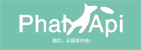# phalapi-入门篇5(数据库操作和Model层)## 1. 基于PDO的notorm进行的数据库操作

phalapi的数据库操作是使用的开源的notorm进行的,notorm是基于PDO链接数据库,在框架内部默认链接的是mysql数据库,如需修改链接其他数据库请修改 /PhalApi/PhalApi/DB/NotORM.php中的getPdo方法:

`````` \$dsn = sprintf('mysql:dbname=%s;host=%s;port=%d',
\$dbCfg['name'],
isset(\$dbCfg['host']) ? \$dbCfg['host'] : 'localhost',
isset(\$dbCfg['port']) ? \$dbCfg['port'] : 3306
);``````

``````<?php
/**
* 数据库接口服务类
*/
class Api_DB extends PhalApi_Api{
public function getRules(){
return array(
'insert' => array(
'id'    => array('name' => 'id', 'require' => true, 'desc' => '用户Id'),
'name'  => array('name' => 'name', 'require' => true, 'desc' => '用户名称'),
'phone' => array('name' => 'phone', 'require' => true, 'desc' => '用户手机号码'),
),
'select' => array(
'id' => array('name' => 'id', 'require' => true, 'desc' => '用户Id'),
),
'update' => array(
'id'    => array('name' => 'id', 'require' => true, 'desc' => '用户Id'),
'name'  => array('name' => 'name', 'require' => true, 'desc' => '用户名称'),
'phone' => array('name' => 'phone', 'require' => true, 'desc' => '用户手机号码'),
),
'delete' => array(
'id' => array('name' => 'id', 'require' => true, 'desc' => '用户Id'),
),
);
}``````

### 1.1 insert接口

``````/**
* 新增表服务
* @return int id 新增列的Id
*/
public function insert(){
\$data = array(                                               //用数组构成需要插入键值一一对应
'id'    => \$this->id,
'name'  => \$this->name,
'phone' => \$this->phone,
);
\$rs   = DI()->notorm->user->insert(\$data);                  //执行数据库操作user代表的是表,返回结果是插入成功的值
return \$rs['id'];                                           //返回插入的id
}``````### 1.2 select接口

``````/**
* 查询
* @return array data 结果集
*/
public function select(){
\$data   = array();
\$data[] = DI()->notorm->user->select('name,phone')->where('id', \$this->id)->fetch();
\$data[] = DI()->notorm->user->select('name,phone')->where('id = ?', \$this->id)->fetchAll();
\$data[] = DI()->notorm->user->select('name,phone')->where('id != ?', \$this->id)->fetchRows();
return \$data;
}``````#### 1.2.1 select方法

select方法主要是用来指定返回值,接受的是一个string他的作用于真正查询语句select和from之间填充,大家如果把select('name,phone') 改为 select('*') 就会得到包括id的所有字段的返回

#### 1.2.2 where方法和排序

where方法是查询中的重要的一个环节

where('id', \$this->id)等同于where('id = ?', \$this->id)

where('id != ?', \$this->id)这种方式只要是为了指定条件大于,等于,小于,不等于

#### 1.2.3 fetch,fetchAll和fetchRows

fetch方法是获取单独的一条数据返回结果是不带下标的数组 ,fetchAll和fetchRows不同在于他们返回的是包含多条数据一个带下标的数组,可以看到在条件一样的情况下第一条和第二条查询出来的结果区别是第二条多了一个0的下标,从此可得到如果是确定返回结果只有一条优先使用fetch,如果是多条结果优先使用fetchAll和fetchRows.

fetchAll和fetchRows还提供了一个功能就是单独执行sql语句

``````\$sql = 'select * from tbl_user where id = :id';
\$params = array(':id' => \$this->id);                 //替换:id为请求参数的id
DI()->notorm->user->queryAll(\$sql, \$params);         //或fetchRows(\$sql, \$params)``````

### 1.3 update接口

``````/**
* 修改
*/
public function update(){
\$data = array(
'name'  => \$this->name,
'phone' => \$this->phone,
);
\$rs   = DI()->notorm->user->where('id', \$this->id)->update(\$data);
if(\$rs === false){
}
}``````

### 1.4 delete接口

``````/**
* 删除
*/
public function delete(){
\$rs   = DI()->notorm->user->where('id', \$this->id)->delete();
if(\$rs === false){
}
}``````

### 1.5 打印sql语句## 2. 使用Model进行数据库操作

### 2.1 传统的Model操作

``````public function getByUserId(\$userId) {
return DI()->notorm->user->select('*')->where('id = ?', \$userId)->fetch();
}``````

``````    \$model = new Model_User();
\$rs = \$model->getByUserId(\$userId);``````

### 2.2 框架自带的Model操作

``````protected function getTableName(\$id) {
return 'user';
}``````

``````public function getByUserId(\$userId) {
return \$this->getORM()->select('*')->where('id = ?', \$userId)->fetch();
}``````

\$this->getORM()相当于DI()->notorm->(getTableName中设置的表名)

``````public function select(){
\$model = new Model_User();
return \$model->getByUserId(\$this->id);
}````````````public function select(){
\$model = new Model_User();
return \$model->get(\$this->id);
}``````